# Electric Power Formula

Electric Power Formula

Electric power is the rate at which energy is transferred to or from a part of an electric circuit. A battery can deliver energy, or a circuit element like a resistor can release energy as heat. For any circuit element, the power is equal to the voltage difference across the element multiplied by the current. By Ohm's Law, V = IR, and so there are additional forms of the electric power formula for resistors. Power is measured in units of Watts (W), where a Watt is equal to a Joule per second (1 W = 1 J/s).

General form:

electric power = voltage difference x current

P = VI

Resistors: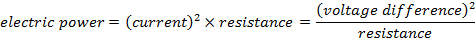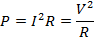P = electric power (W)

V = voltage difference (V = J/C)

I = electric current (A = C/s)

R = resistance (Ω = V/A)

Electric Power Formula Questions:

1) If the battery of a cell phone operates at 12.0 V, and it has to deliver a current of 0.9 A while playing music, what is the power required?

Answer: The power required from the battery can be found using the electric power formula:

P = VI

P = (12.0 V)(0.9 A)

P = (12.0 J/C)(0.9 C/s)

P = 10.8 J/s

P = 10.8 W

The power required from the battery of the phone is 10.8 W.

2) A resistor with a 24.0 V potential difference across it is radiating heat. The thermal energy is being generated at a rate of 16.0 W. What is the resistance value?

Answer: The resistance value can be found by rearranging one of the forms of the electric power formula. The form that is applicable relates power, voltage, and resistance: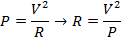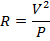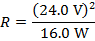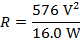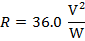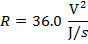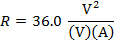R=36.0 V/A

R=36.0 Ω

The resistance value is 36.0 Ω.